fglynn

•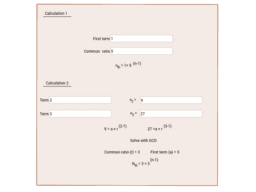Geometric sequence calculator

Activity

fglynn

•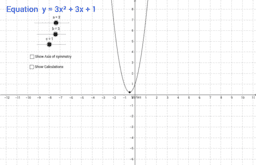Axis of symmetry and coefficients of a quadratic

Activity

fglynn

•Sine and Cosine Transformations

Activity

fglynn

•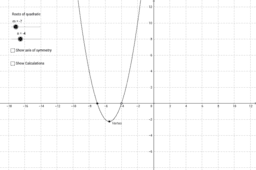Axis of symmetry and roots of a quadratic function

Activity

fglynn

•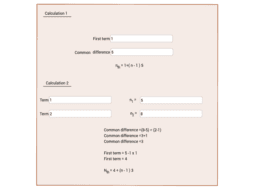Arithmetic sequence calculator

Activity

fglynn

•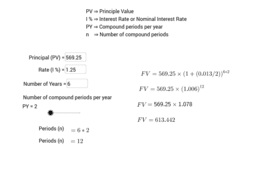Compound Interest Calculator

Activity

fglynn

•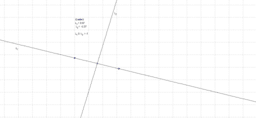Activity

fglynn